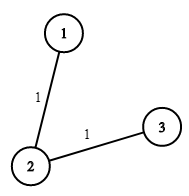## 题目描述：

LeetCode 1168. Optimize Water Distribution in a Village

There are `n` houses in a village. We want to supply water for all the houses by building wells and laying pipes.

For each house `i`, we can either build a well inside it directly with cost `wells[i]`, or pipe in water from another well to it. The costs to lay pipes between houses are given by the array `pipes`, where each `pipes[i] = [house1, house2, cost]` represents the cost to connect `house1` and `house2` together using a pipe. Connections are bidirectional.

Find the minimum total cost to supply water to all houses.

Example 1:```Input: n = 3, wells = [1,2,2], pipes = [[1,2,1],[2,3,1]]
Output: 3
Explanation:
The image shows the costs of connecting houses using pipes.
The best strategy is to build a well in the first house with cost 1 and connect the other houses to it with cost 2 so the total cost is 3.
```

Constraints:

• `1 <= n <= 10000`
• `wells.length == n`
• `0 <= wells[i] <= 10^5`
• `1 <= pipes.length <= 10000`
• `1 <= pipes[i], pipes[i] <= n`
• `0 <= pipes[i] <= 10^5`
• `pipes[i] != pipes[i]`

## 解题思路：

Kruskal（最小生成树）

Kruskal的基本思路是贪心（Greedy）。利用边集求最小生成树。首先对边集edges排序，然后遍历edges，利用并查集(Union-Find Set)对边的端点进行合并。若两端点不在同一个集合中，则将两端点进行merge，并记录权重。

## Python代码：

``````class Solution(object):
def minCostToSupplyWater(self, n, wells, pipes):
"""
:type n: int
:type wells: List[int]
:type pipes: List[List[int]]
:rtype: int
"""
for x, w in enumerate(wells):
pipes.append((x + 1, n + 1, w))
pipes.sort(key = lambda k: k[-1])
parents = {i:i for i in range(n+2)}
def find(i):
while i != parents[i]:
i = parents[i]
return i
ans = 0
for x, y, z in pipes:
x = find(x)
y = find(y)
if x != y:
ans += z
parents[x] = y
return ans
``````

Pingbacks已关闭。